# Jointly Training Large Autoregressive Multimodal Models

This is my reading note for Jointly Training Large Autoregressive Multimodal Models. This paper proposes a multimodality model for generating images. The paper is not just dilution based method but instead auto regressive method.it argues to initialize the model from the weight of frozen models.

we propose to combine two autoregressive decoder-only architectures. Our primary image-text model is (p. 1)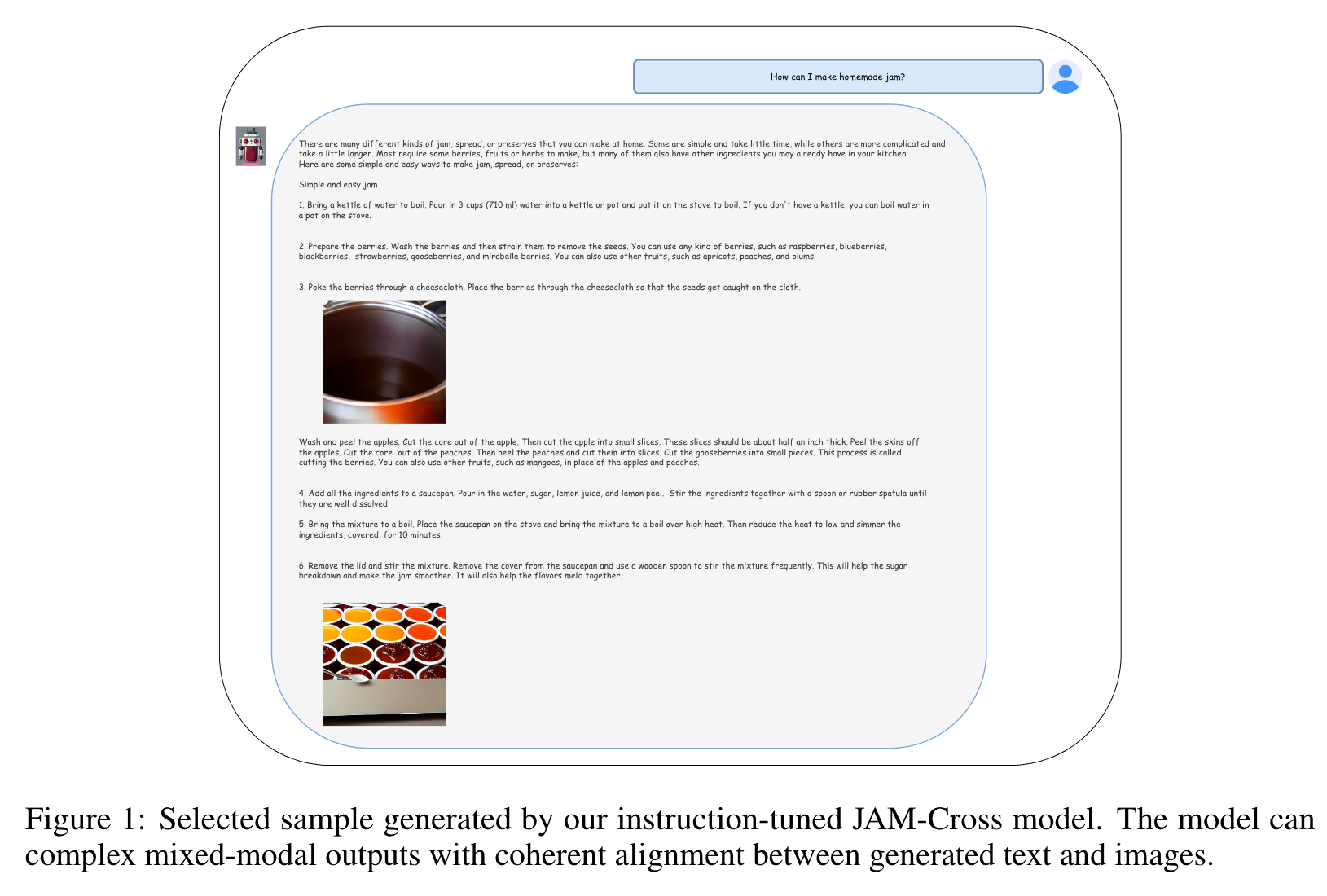# Introduction

To address this gap, we present the Joint Autoregressive Mixture (JAM) framework, a modular approach that systematically fuses existing text and image generation models. We also introduce a specialized, data-efficient instruction-tuning strategy, tailored for mixed- modal generation tasks. (p. 1)

To achieve this objective, we conduct a comprehensive empirical investigation into the fusion of two specialized autoregressive, decoder-only, large transformer models, each designed for unique tasks (one for text-to-image and a text only model). We introduce a set of methods under the umbrella of the Joint Autoregressive Mixture (JAM) framework. In building this framework, we take advantage of the inherent architectural compatibility of autoregressive text-to-image models with LLMs, allowing us to do deep model fusion and joint training in ways which would otherwise not be possible. (p. 1)

Concurrently to developing diffusion-based generative models, significant steps have been made by autoregressive token models (Esser et al., 2021; Gafni et al., 2022). These models encode images into a discrete latent space (Van Den Oord et al., 2017) and can be processed as a standard sequence-to-sequence modeling task, enabling the borrowing of techniques used from Large Language Models. A critical element that has been found beneficial in boosting text-to-image generative models is retrieval augmentation (p. 9)

# Proposed Method

CM3leon (Yu et al., 2023), trained on 2.4T image-text caption tokens. In contrast, using the same architecture, our LLM (Molybog et al., 2023) has been trained on 1.4T text tokens. In the first stage (Sect. 2.1), we first combine and align the models. In the second stage (Sect. 2.2), we explore new directions for instruction tuning focused on interleaved image-text generation. (p. 2)

## CONTINUED PRETRAINING

This composite model is fine-tuned using a hybrid dataset comprising both text-only and image-text samples within our continued pretraining phase. (p. 2)

### MODEL MERGING

The concept of model merging has been previously utilized to combine models that share identical optimization trajectories (Kaddour et al., 2022), or models that are trained on identical datasets but have independent optimizations (for instance, Matena & Raffel (2022); Wortsman et al. (2022); Ainsworth et al. (2022)). A consistent approach across these studies is to combine models without any training. Our approach diverges from this convention; we view the merged model as a powerful initialization for subsequent training on mixed-modal data. The weights of the averaged model are defined as: (p. 2)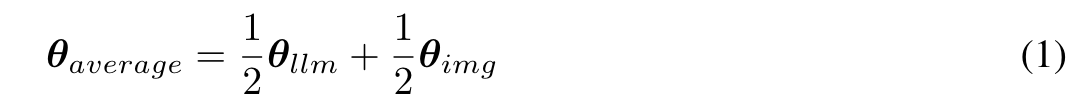In this study, we explore weights merging specifically to multimodal decoder-only large transformer models, and notably, on an unprecedented scale, involving models trained on trillions of tokens from diverse datasets. In the following sections, we refer to our average model as JAM-Uniform. (p. 2)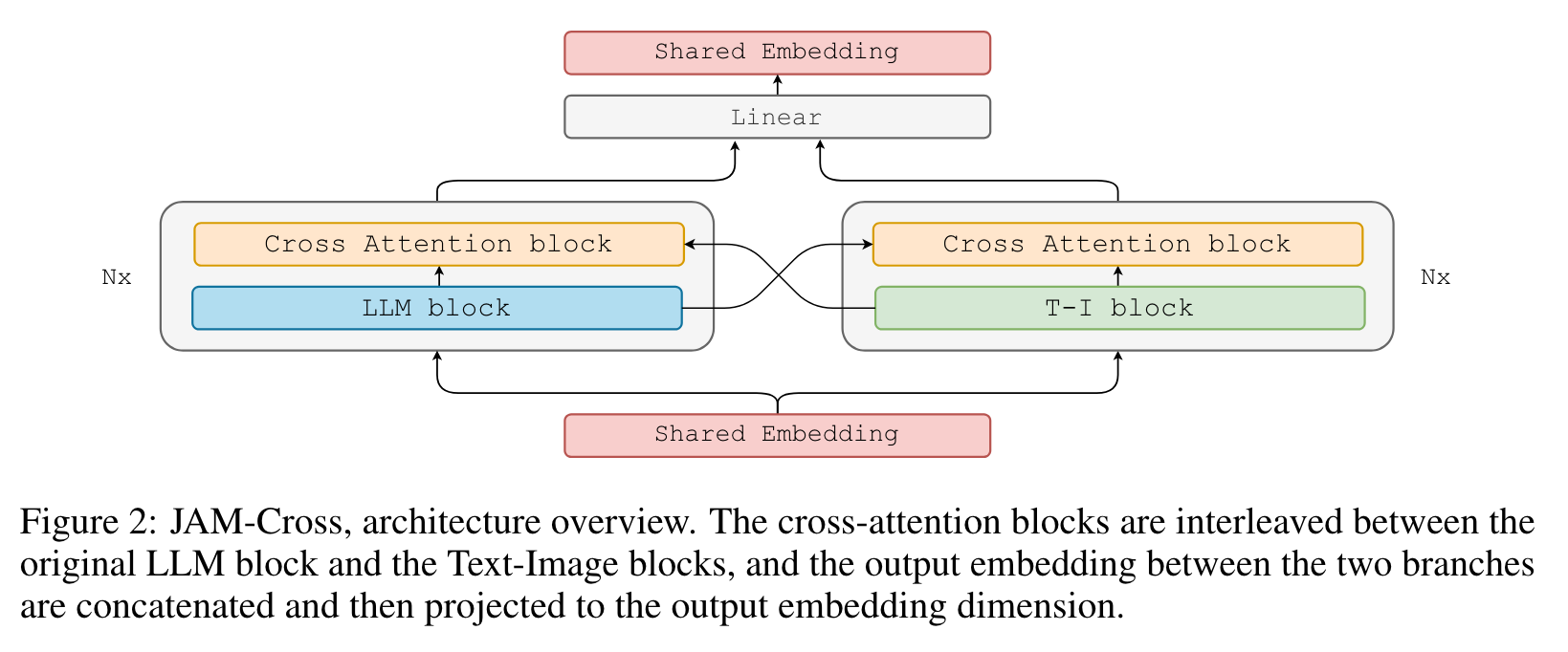### WIDTH CONCATENATION

Our second approach employs the pretrained weights to initialize a wider architecture. Our new model has hidden dimensions djoint = 8192, which is doubled with respect to one of the two original models dllm = dimg = 4096. We keep the same number of layers of the original architectures. The resulting architecture has 26B parameters, initialized starting from the pretrained weights of our backbones. The token embedding input/output projections and the learned positional embeddings of the two initial models are concatenated on the hidden dimension. The attention weights (e.g query projection) Wq,combined ∈ Rdjoint×djoint are initialized as: (p. 3)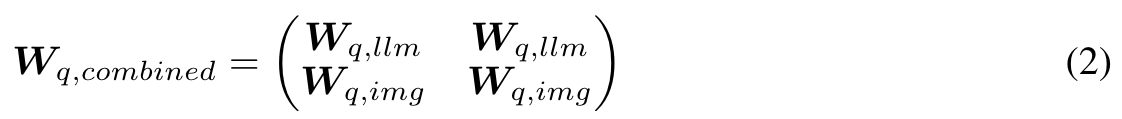All the other weights (FFNs and output projections) are initialized following the same logic. We also experiment with slight variations of the approach: (p. 3)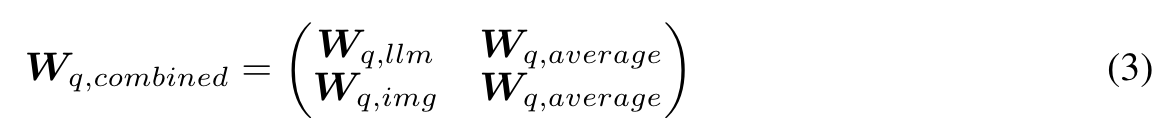### CROSS MODEL FUSION

We propose to embed cross-attention layers between the foundational models to facilitate seamless information interchange while preserving the original models’ knowledge. Given two decoder-only transformers models Tllm and Timg, we introduce a bi-directional cross-attention mechanism that enables the layers of one model to attend to the corresponding layer’s output of the other model. (p. 3)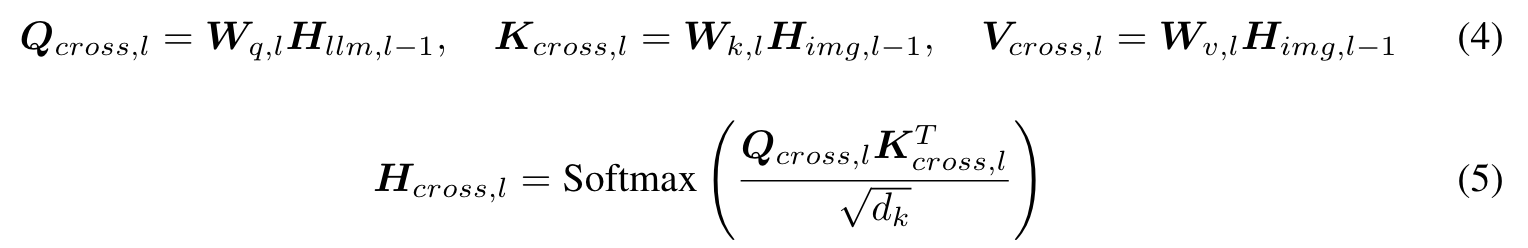We use a shared input-output projection layer, initializing the weights of the text tokens from the LLM input embedding and the weights of the image tokens from the image-text model. We insert a new linear projection layer that takes the concatenation of the two model’s output embeddings as input. (p. 3)

## MULTIMODAL CONVERSATIONAL INSTRUCT TUNING

In this work, we study instruction tuning tailored to interleaved image-text generation. We collect a small and curated mixed-modal dataset to teach our JAM model to support textual explanations with coherent images. Since in the first stage, the model has been trained on image-text captions and text-only data; we train on interleaved image-text data during this phase. (p. 4)

# EXPERIMENTS

## Tokenizers

For images, we use the VQ-VAE tokenizer from Gafni et al. (2022). The image resolution is set to 256 × 256, 1024 tokens represent each image, and the vocabulary has a size of 8192. Our text tokenizer is the same that have been used to train the two parent models, trained over the Zhang et al. (2022) data for text. We introduce the additional <break> token used by CM3leon to identify a modality break. (p. 4)

## Objective

In all our experiments, we employ the CM3 objective introduced in Aghajanyan et al. (2022); this objective accepts the original sequence as input or transforms it into an infilling instance by masking specific spans and relocating them to the end. Then, the model is optimized for minimizing the standard autoregressive loss − log p(xinput). (p. 4)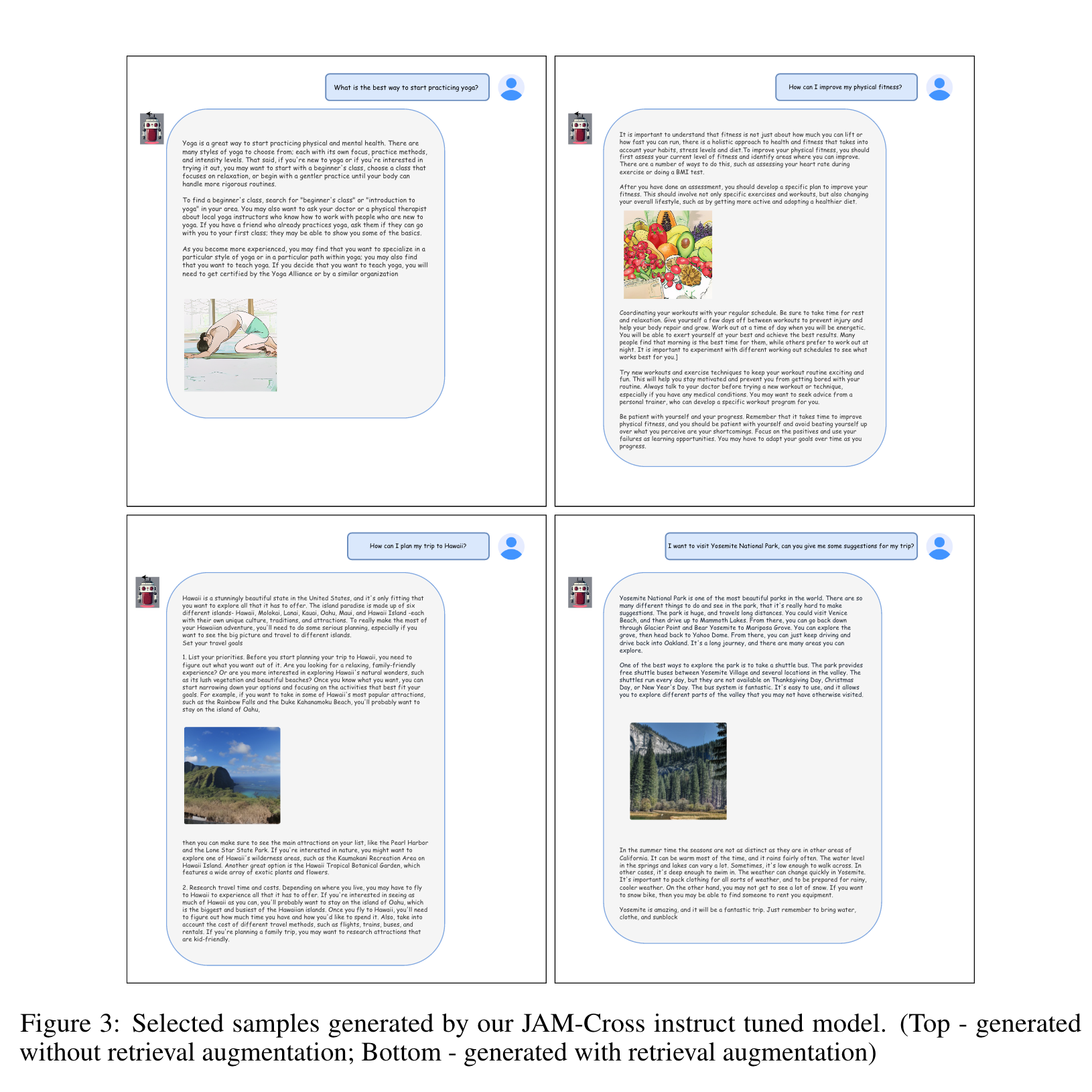## Decoding Strategies

We implement a mixed-modal decoding strategy for our interleaved generation. The model starts generating text tokens until a modality <break> token is detected, then an image is sampled, and the generation continues until a <eos> token is sampled. (p. 6)

We also employ classifier- free guidance (CFG (Gafni et al., 2022)) for sampling images. (p. 6)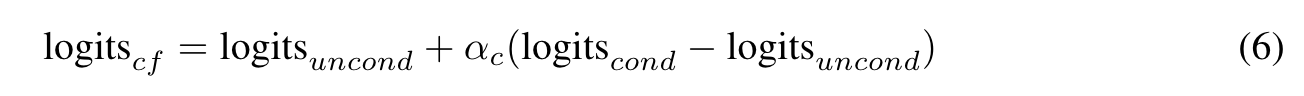where logits_cond = T (ty tx) and logits_uncond = T (ty| < mask >); T represent the transformer model, < mask > represent the absence of the input text, tx are the conditional input tokens, ty are the output tokens and αc is the scaling factor for CFG (p. 6)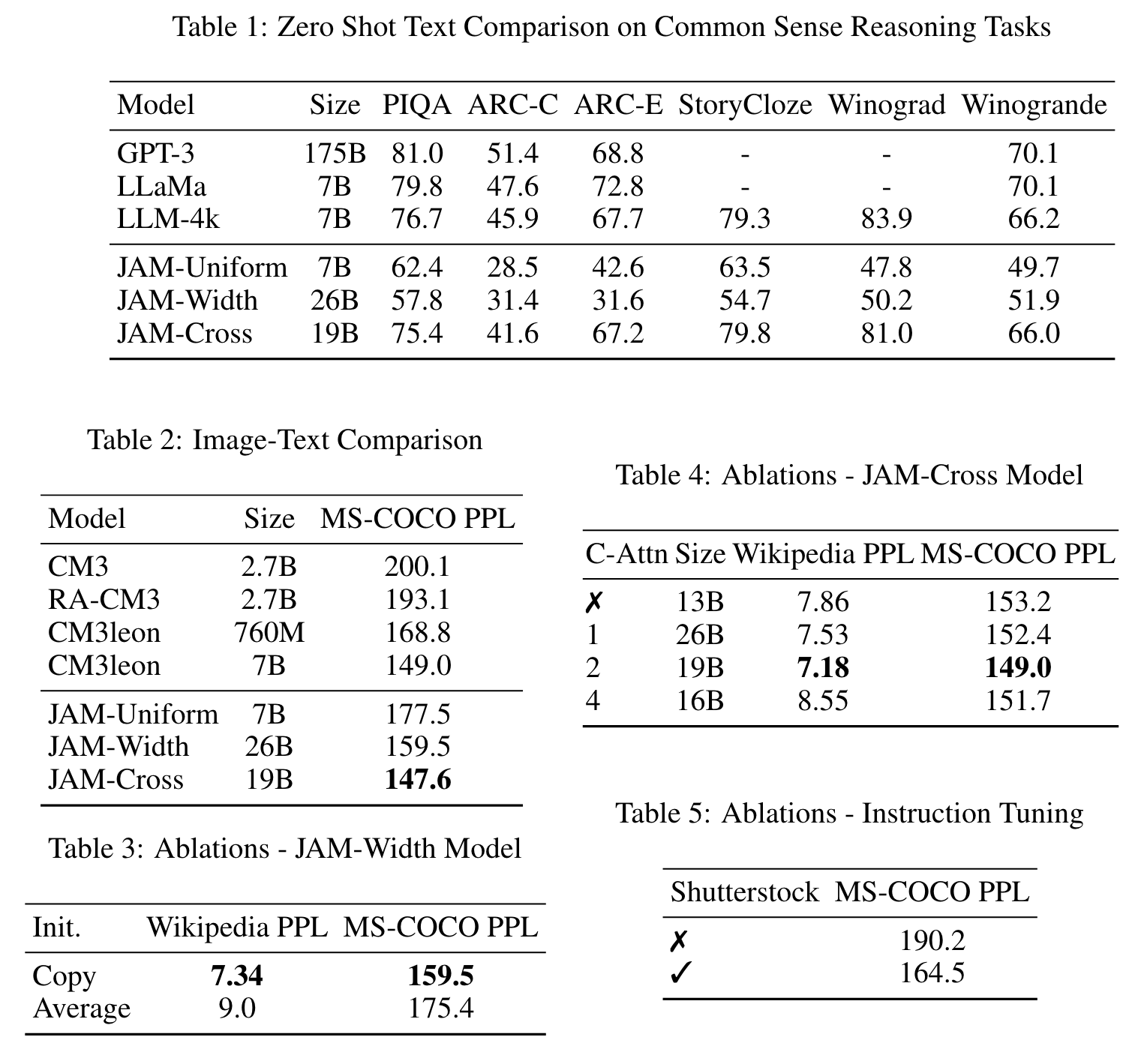# ABLATION STUDY

Results (Table 3) show that copying the weights is more effective than averaging them to retain the original model capabilities. (p. 9)

The results indicate the importance of using pretraining data mixed in the instruction tuning procedure to preserve the MS-COCO PPL. We do not report WikiHow PPL since analyzing the models shows that it doesn’t correlate with generation quality similarly to Zhou et al. (2023) (p. 9)

Written on July 28, 2023